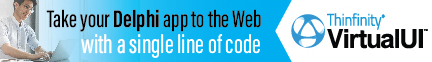DelphiBasicsInfinityConstant Floating point value of infinite size Math unit
 const Infinity =  1.0 / 0.0;
Description
The Infinity constant is a special floating point number, marked as infinite in size.

It may be assigned and calculated upon, but use IsInfinite for comparisons.
Related commands
 IsInfinite Checks whether a floating point number is infinite IsNaN Checks to see if a floating point number holds a real number NaN Not a real number
Author linksDownload this web site as a Windows program.

 Example code : Illustrate use of Infinity var   float1, float2 : single; begin   float1 := Infinity;   float2 := 23;   ShowMessage('float1 = '+FloatToStr(float1));   ShowMessage('float2 = '+FloatToStr(float2));   ShowMessage('float1 - float2 = '+FloatToStr(float1 - float2));   ShowMessage('-float1 = '+FloatToStr(-float1)); end; Show full unit code float1 = INF   float2 = 23   float1 - float2 = INF   -float1 = -INF
 Delphi Programming © Neil Moffatt 2002 - 2020. All rights reserved.  |  Home Page